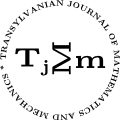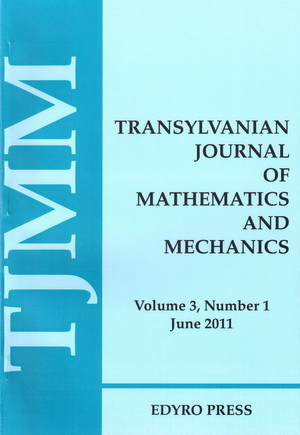ISSN: 2067-239X
ISSN(on-line): 2067-239X

Indexed in:
Mathematical Reviews
Zentralblatt MATH
EBSCO

Front cover# Volume 9 (2017), Number 1

## Calculations of Some Physical Entities of the Universe Expansion Using Its Symplified Mathematical Model

### Author(s): MALVINA BAICA and MIRCEA CARDU

Abstract: In this paper we consider and solve the following problems related to the Universe Expansion (UE): 1.1. The determination of the mathematical relations for the velocity of the UE as a function of time. 1.2. The calculation of “the Hubble constant” value as a function of time.

## Inequalities for Riemann-Stieltjes Integral with Applications for Selfadjoint Operators and Quantum Divergence Measures

### Author(s): S.S. DRAGOMIR

Abstract: Some inequalities for Riemann-Stieltjes integral that provide weighted reverses of Jensen’s inequality are obtained. Applications for functions of selfadjoint operators and quantum $f$-divergence measures are also given.

## On ${H}_{3}\left(1\right)$ Hankel Determinant for Univalent Functions Defined by Using $q$-Derivative Operator

### Author(s): ZELIHA KARAHUSEYIN, ŞAHSENE ALTINKAYA and SIBEL YALÇIN

Abstract: In this work, we introduce a new subclass of univalent functions deﬁned by using $q$-derivative operator. Moreover, for functions belonging to this class, we establish third Hankel determinant denoted by ${H}_{3}\left(1\right)$.

## On Co-Ordinated Ostrowski and Hadamard’s Type Inequalities for Convex Functions II

Abstract: In this article, we establish various inequalities for some diﬀerentiable mappings that are linked with the illustrious Hermite-Hadamard and Ostrowski integral inequality for convex functions of several-variables on the co-ordinates.The generalized integral inequalities contribute some better estimates than some already presented.

## Correlation Analysis of Consumer Price Indices by Multiple Regression

### Author(s): ANAMARIA POPESCU

Abstract: The analysis of these correlations between the consumer price indices, by multiple regression, supplements the information and the conclusions drawn by the implementation of models of the regression type simple. Additional information obtained through the use of multiple regression represents a support additional information for decision-makers and analysts. This article describes a correlation between the annual index of consumer prices, total annual index of consumer prices of foodstuffs, annual index of consumer prices of non-food goods and the annual index of consumer prices of services, by means of a regression model in multiple. The model explains the influence of the three types of consumption on the evolution of the annual index of consumer prices total and allows the creation of forecasts.

## Certain Classes of Analytic Functions Involving A Family Of Generalized Differential Operators

### Author(s): C. SELVARAJ, G. THIRUPATHI and E. UMADEVI

Abstract: In this paper, we deﬁne a new class of analytic function using generalized differential operator. Coefficient inequalities, sufficient condition, distortion theorems, radii of starlikeness, convexity and close - to - convexity results are obtained.

## Oscillation Theorems For Second Order Quasi-linear Difference Equations with Linear Neutral Terms

### Author(s): S. SELVARANGAM, S.A. RUPADEVI and E. THANDAPANI

Abstract: This paper studies the oscillatory behavior of solutions of second order quasi-linear difference equation with several neutral terms of the from $\Delta \left({a}_{n}{\left(\Delta \left(\sum _{i=1}^{m}{p}_{in}{x}_{{\tau }_{i}\left(n\right)}\right)\right)}^{\alpha }\right)+{q}_{n}f\left({x}_{\sigma \left(n\right)}\right)=0,\phantom{\rule{2.77695pt}{0ex}}n\ge {n}_{0},$ where $\alpha$ is a ratio of odd positive integers. Some new sufficient conditions are presented which include several existing results. Examples are provided to illustrate the main results.

## Some Integral Inequalities for Twice Differentiable Functions

### Author(s): ERHAN SET, ABDURRAHMAN GÖZPINAR and İMDAT İŞCAN

Abstract: In this paper a general integral identity for twice differentiable convex functions is derived. We establish some new Hermite Hadamard’s type inequalities for functions whose absolute values of derivatives are convex.

## Identities for Hypergeometric Integrals and Euler Sums

### Author(s): ANTHONY SOFO

Abstract: We provide an explicit analytical representation for a number of logarithmic and hypergeometric function integrals in terms of the polygamma function and other special functions. The integrals in question will be associated with harmonic numbers of positive terms. A few examples of integrals will be given an identity in terms of some special functions including the Riemann zeta function.

## On Nonlinear Caputo Fractional $q$-Difference Boundary Value Problems With Multi-Point Conditions

### Author(s): WENGUI YANG, QINGBO ZHAO and CHAOWU ZHU

Abstract: In this paper, we investigate a class of nonlinear boundary value problems of Caputo type fractional $q$-difference equations supplemented with nonlocal multi-point conditions. By making use of some well known tools of the ﬁxed point theory, the existence and uniqueness of solutions for the proposed problems are established. As applications, two examples are presented to demonstrate the effectiveness of the obtained results.

Abstract: In this paper an attempt has been made to study the wave propagation through a one dimensional elastic solid in the frame work of Eringen’s nonlocal elasticity using the concept of fractional calculus. The fractional differential equation corresponding to the axial harmonic wave propagation through a one dimensional elastic bar of infinite length with uniform cross section is obtained. The dynamic equation for the harmonic wave propagation in terms of Caputo fractional derivatives of various orders $\alpha$ has been derived. The dispersive equation for an axial wave is obtained in terms of fractional order $\alpha$ and a parameter ${\beta }_{2}$, which accounts the eﬀect of nonlocal long range interactions. The effect of wave number on this ratio is studied numerically for different values of the fractional order and the nonlocal parameters.